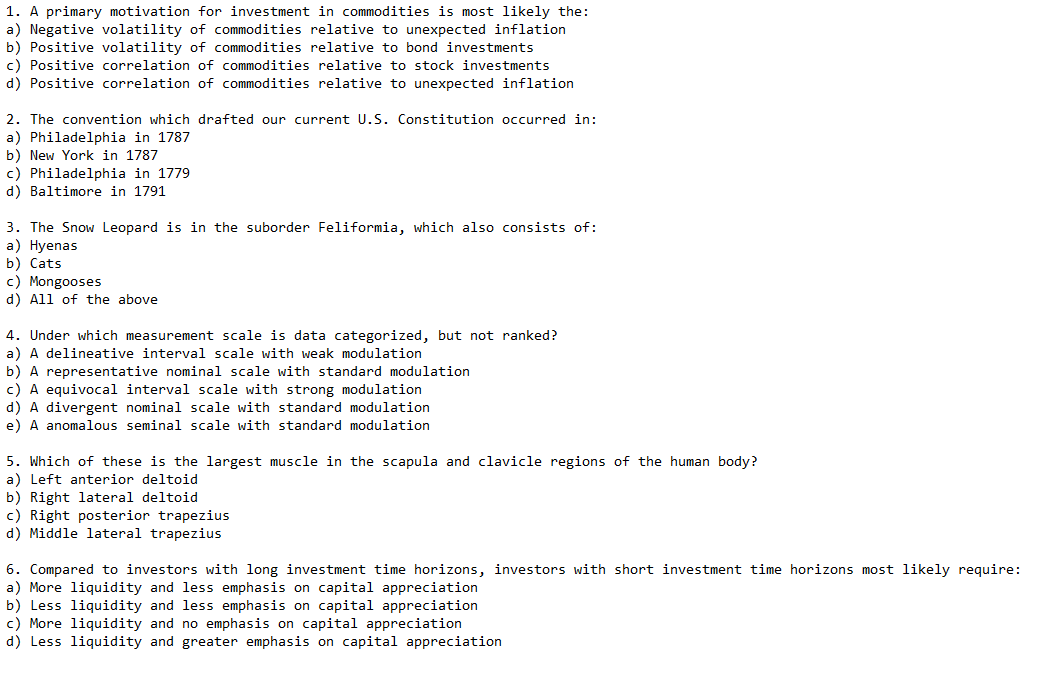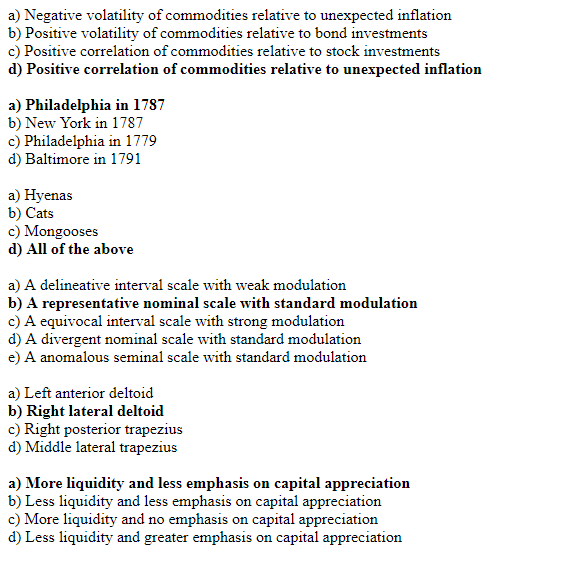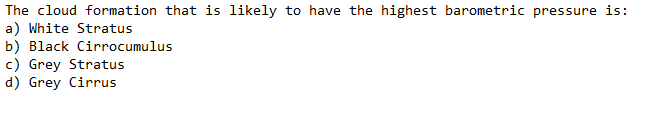# matt-stam/calcipher

Calculates the best possible answer for multiple-choice questions using techniques to maximize accuracy without any other outside resources or knowledge.
Java HTMLFetching latest commit…
Cannot retrieve the latest commit at this time.
Type Name Latest commit message Commit time
Failed to load latest commit information.src/stam/mainpackage.classpath.gitignore.projectAnswerKey.htmlExampleQuestions.txtLICENSEREADME.md

# Calcipher

Calcipher is a Java program that calculates the best possible answer for multiple-choice questions using techniques to maximize accuracy without any other outside resources or knowledge.

### Example Questions:### Result:### How To Use

Enter in a text file with the questions you want analyzed. The program will determine the best possible answers for each question. Those answers are bolded in an HTML file.

### Methodology

In theory, guessing on a 4 answer multiple choice test would yield a result of 25% correct. However, we can increase our accuracy if we exploit the techniques that creators typically use to design these tests. There are multiple ways we can reverse-engineer these techniques and use them to bolster the amount of questions we can correctly guess.

One of the most powerful of these techniques is to look at word occurrences between each question's answers. When false answer choices are designed, they are made with slight deviations from the correct choice. In these false answers, there is often a part of the answer that is correct combined with part of the answer that is incorrect. This gives each question multiple answers that seem viable, which should make it harder to guess on. However, if we look for these types of patterns, we can use them to guess the correct answer.

For example:Assuming you have no knowledge meteorology, you should have a 25% chance of guessing the correct response. But we can use our knowledge of how these questions are created to maximize our chances of being correct. There is two traits to look at in each choice, the color and the type. Of all the questions, the only response with repeated traits is option C. The color choice is the same as option D, and the type is the same as option A. Because no other option has this much in common with the rest, the best possible guess is always C.

We can combine this method with others that will help improve our accuracy even more. We can give biases to "None of the above, All of the above, etc" type questions, which have ~52% chance of being correct. We can look at answer length, exploiting the fact that the correct choices have to be unequivocally correct, which lends itself to expressive language, and thus are typically longer answers.

Each one of these factors gets its own bias which changes how much the result of each method should weigh. These biases are summed up to determine which answer is the best possible choice to maximize accuracy.

Calcipher does all of this on a large scale. It takes in any multiple choice test (which can have any number of options) and outputs the best possible guesses by bolding them in a HTML file.

You can’t perform that action at this time.